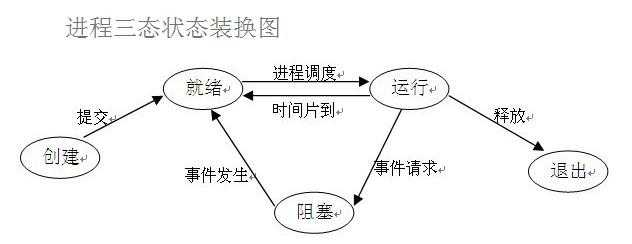# 复习打卡--0823 队列和多进程

Python的Queue模块中提供了同步的、线程安全的队列类，包括FIFO（先入先出)队列QueueLIFO（后入先出）队列LifoQueue，和优先级队列PriorityQueue。这些队列都实现了锁原语，能够在多线程中直接使用。可以使用队列来实现线程间的同步。

queue.Queue(maxsize=0)  maxsize默认为0，不设置或设置为负数时，表示可接受的消息数量没有上限。

Queue.join()       实际上意味着等到队列为空，再执行别的操作

Queue.put(item,block=True, timeout=None)     写入队列，block=True,Timeout=3 意味着等待3s，队列仍没有位置就报错；block=False 意思是不等待，队列如果满了直接报错；

Queue.get(block=True, timeout=None)    获取队列，block和timeout参数说明同上put

Queue.get_nowait()       相当于Queue.get(block=False)，不等待

Queue.put_nowait()       相当于Queue.put(block=False)，不等待

Queue.qsize()     返回队列的大小

Queue.empty()    如果队列为空，返回True,反之False

Queue.full()         如果队列满了，返回True,反之False，Queue.full 与 maxsize 大小对应

```from  queue import Queue,LifoQueue,PriorityQueue

#先入先出队列
q1=Queue(masize=2)

q1.put(1)
q1.put(2)
print(q1.full())  # 判断队列是否已满，返回True
print(q1.qsize())  # 返回当前队列元素的个数
q1.put(3,block=False)  # 向队列中加入值，不等待，直接报错。
q1.get()
q1.get()
print(q1.empty())  # 判断队列是否为空，返回True

```from threading import Thread,Lock
from queue import Queue

q=Queue(maxsize=2)
num=0
q.put(num)

def work1():
global num
for i in range(1000000):
q.get()  #读取队列中的值
num+=1
q.put(num)  #加入队列

def work2():
global num
for i in range(1000000):
q.get()
num+=1
q.put(num)  #加入队列

def main():
t1.start()
t2.start()
t1.join()
t2.join()
print(num)

if __name__==‘__main__‘:
main()```

LifoQueue 后入先出队列: 继承了Queue，所有方法用法与Queue一样，仅仅是取出的顺序发生变化。

PriorityQueue 优先级队列： q=PriorityQueue ()     q.put((1,22))  以元祖形式添加元素，前面为优先级，后面为元素。优先级越低，越先取出。

1.什么是进程？

2.进程状态介绍在了解其他概念之前，我们首先要了解进程的几个状态。在程序运行的过程中，由于被操作系统的调度算法控制，程序会进入几个状态：就绪，运行和阻塞。

当进程已分配到除CPU以外的所有必要的资源，只要获得处理机便可立即执行，这时的进程状态称为就绪状态。

（2）执行/运行（Running）状态当进程已获得处理机，其程序正在处理机上执行，此时的进程状态称为执行状态。

（3）阻塞(Blocked)状态正在执行的进程，由于等待某个事件发生而无法执行时，便放弃处理机而处于阻塞状态。引起进程阻塞的事件可有多种，例如，等待I/O完成、申请缓冲区不能满足、等待信件(信号)等。

事件请求：input、sleep、文件输入输出、recv、accept等

事件发生：sleep、input等完成了

时间片到了之后有回到就绪状态，这三个状态不断的在转换。

3.进程，线程对比

功能：

• 进程，能够完成多任务，比如 在一台电脑上能同时运行多个软件

• 线程，能够完成多任务，比如一个QQ中的多个聊天窗口

定义：

• 进程是系统进行资源分配和调度的一个独立单位

• 线程是进程的一个实体，是CPU调度和分配的基本单位，它是比进程更小的能独立的基本单位，线程自己基本上不拥有系统资源，只拥有一点在运行中必不可少的资源（如程序计数器，一组寄存器和栈），但是它可与同属一个进程的其他线程共享所拥有的全部资源

区别：

• 一个程序至少有一个进程，一个进程至少有一个线程

• 线程的划分尺度小于进程（资源比进程少），使得多线程程序并发性高

• 进程在执行过程中拥有独立的内存单元，而多个线程共享内存，从而极大地提高了程序的运行效率

• 线程不能够独立执行，必须依存在进程中

• 可以将进程理解为工厂中的一条流水线，而线程就是流水线上的工人

优缺点：

• 线程执行开销小，但不利于资源的管理和保护；进程正相反

```from multiprocessing import Process
import time

def work1():
for i in range(6):
time.sleep(1)
print(f‘第{i+1}次浇花中‘)

def work2(name):
for i in range(5):
time.sleep(1)
print(f‘第{i+1}次{name}打墙中‘)

#windows下不能直接像下面这样引用，新建进程时会先import 进程.py原文件---执行--导入--执行--，陷入无限递归中报错。
# 一定要在main下面使用，这样即使导入进程脚本，也会因为运行的不是原脚本文件而不再继续执行
#linux/mac上不会报错，引入机制不同。
# p1=Process(target=work2,args=(‘musen‘,))
# p1.start()
# work1()

def main():
t1 = Process(target = work2 , args = (‘musen‘ ,))  # Thread(target=work2,kwargs={‘name‘:‘musen‘}) 这种方式传参
t1.start()
work1()

#单任务，多进程
if __name__ == ‘__main__‘:
main()```

```from multiprocessing import Process

class MyProcess(Process):
def run(self):
with open(‘1.txt‘,‘a‘,encoding = ‘utf8‘) as f:
for i in range(2):
f.write(‘python\n‘)

def main():
p_list=[]
for i in range(3):
p=MyProcess()
p.start()
for j in p_list:
j.join()

if __name__=="__main__":
main()```

p=multiprocessing.Process(group=None, target=None, name=None, args=(), kwargs={}, daemon=None)

1. group 参数未使用，值始终为None，大部分情况用不到
2. target 表示调用对象，即子进程要执行的任务
3. args 表示调用对象的位置参数元组，args=(1,2,‘egon‘,)
4. kwargs 表示调用对象的字典,kwargs={‘name‘:‘egon‘,‘age‘:18}
5. name 为子进程的名称

Process创建的实例方法：

1. p.start()：启动进程，并调用该子进程中的p.run()
2. p.run(): 进程启动时运行的方法，正是它去调用target指定的函数，我们自定义类的类中一定要实现该方法
3. p.terminate(): 强制终止子进程p，不会进行任何清理操作，如果p创建了子进程，该子进程就成了僵尸进程，使用该方法需要特别小心这种情况。如果p还保存了一个锁那么也将不会被释放，进而导致死锁
4. p.is_alive(): 判断进程是否活着，如果p仍然运行，返回True
5. p.join([timeout]): 是否等待子进程结束，（强调：是主进程处于等的状态，而p是处于运行的状态）。timeout是可选的超时时间，需要强调的是，p.join只能join住start开启的进程，而不能join住run开启的进程

Process创建的实例属性：

1. p.daemon：默认值为False，如果设为True，代表p为后台运行的守护进程，当p的父进程终止时，p也随之终止，并且设定为True后，p不能创建自己的新进程，必须在p.start()之前设置
2. p.name:进程的名称，默认为Process-N，N为从1开始递增的整数
3. p.pid：进程的pid，进程号，也可以通过os.getpid()获取当前进程号
4. p.exitcode:进程在运行时为None、如果为–N，表示被信号N结束(了解即可)
5. p.authkey:进程的身份验证键,默认是由os.urandom()随机生成的32字符的字符串。这个键的用途是为涉及网络连接的底层进程间通信提供安全性，这类连接只有在具有相同的身份验证键时才能成功（了解即可）

```import os
from multiprocessing import Process

num=0

def work1():
global num
for i in range(100000):
num+=1
print(F‘进程号{os.getpid()}‘,num)

def work2():
global num
for i in range(100000):
num+=1
print(F‘进程号{os.getpid()}‘,num)

def main():
p1=Process(target=work1)
p2=Process(target=work2)
p1.start()
p2.start()
p1.join()
p2.join()
print(‘主进程num值‘,num)

if __name__==‘__main__‘:
main()  #返回 进程号11844 100000，   进程号2224 100000，主进程num值 0```

```from multiprocessing import Process,Lock

def work3(metux):
metux.acquire()
with open(‘1.txt‘ , ‘a‘ , encoding = ‘utf8‘) as f :
for i in range(3) :
f.write(‘python\n‘)
metux.release()

def work4(metux):
metux.acquire()
with open(‘1.txt‘ , ‘a‘ , encoding = ‘utf8‘) as f :
for i in range(3) :
f.write(‘java\n‘)
metux.release()

def main():
metux=Lock()
p1=Process(target=work3,args = (metux,))
p2=Process(target=work4,args = (metux,))
p1.start()
p2.start()
p1.join()
p2.join()

if __name__==‘__main__‘:
main() ```

(0)
(0)